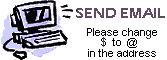W I N I O N O W
Science Trivia #4
The Contest Center
Wappingers Falls, NY 12590
 W I N I O N O W

D01:   What solid shape has 12 identical faces?   [Answer]
D02:   What is the process where a substance is hardened by sudden immersion in a liquid?   [Answer]
D03:   What are the principal metals used in an alnico magnet?   [Answer]
D04:   What is the large central root of many plants called?   [Answer]
D05:   How many roots do human teeth have?   [Answer]
D06:   What is the high-altitude high-speed wind that blows from west to east?   [Answer]
D07:   What is a cold northern desert called?   [Answer]
D08:   What solid shape has the largest volume for a given surface area?   [Answer]
D09:   What natural element has the largest atom?   [Answer]
D10:   What is the densest metal?   [Answer]
D11:   What is the line separating the Northern and Southern hemispheres?   [Answer]
D12:   Is the time for the earth to make one rotation around its axis less than, equal to, or more than 24 hours?   [Answer]
D13:   What is the fatty coating on sheep's wool?   [Answer]
D14:   Which of the following numbers is not prime: 109, 119, 139, 149?   [Answer]
D15:   What temperature is absolute zero on the Fahrenheit scale?   [Answer]
D16:   What is the main artery leaving the heart?   [Answer]
D17:   How does the mass of the moon compare to the mass of the earth?   [Answer]
D18:   Where is the plantar region found?   [Answer]
D19:   What metal is the most chemically reactive?   [Answer]
D20:   How much wood does an adult beaver eat in an average year?   [Answer]Send us an email to submit answers to these questions, to ask for help, or to submit new questions. Be sure to change the \$ to an @ in our email address.